|

# 设计师用新材料晒出上海创意 第十一届“晒上海”设计展开幕2020年1月4日，第十一届“晒上海”设计展在被称为创新体验零售与沉浸艺术融合的智能空间——TX 淮海｜年轻力中心开幕。本届“晒上海”以【小强】为主题，共展出20多位设计师的23件设计作品，这些作品均采用杜邦公司的特卫强（Tyvek）材料作为主材，而设计的作品则聚焦少儿用品。

【作品名录】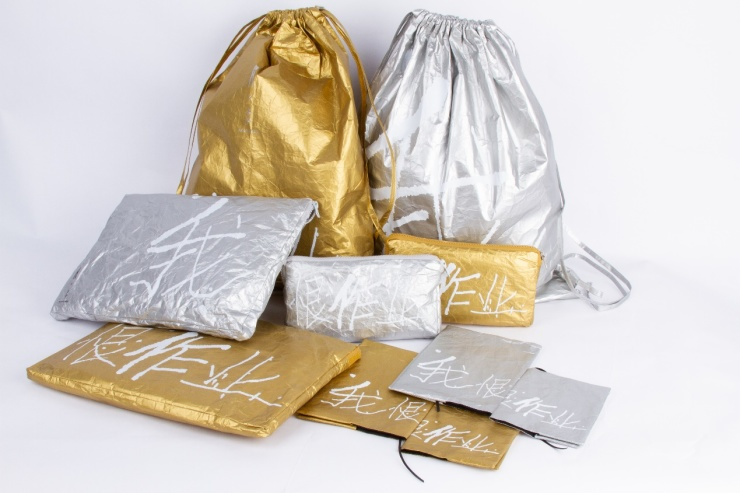1我恨作业 I hate homework （销售产品）

（尺寸约：大300*400，中300*200，小250*100，3套）2 Tyvek DIY儿童防过敏腰带 Tyvek DIY Kid’s Belt

（尺寸约：1000*50，3根）

3 Tyvek 学童逃生绳 Tyvek Escape Rope(缺图)

（尺寸约：3000*30，两根，吊挂，下坠吊环，同图，需展览公司购买）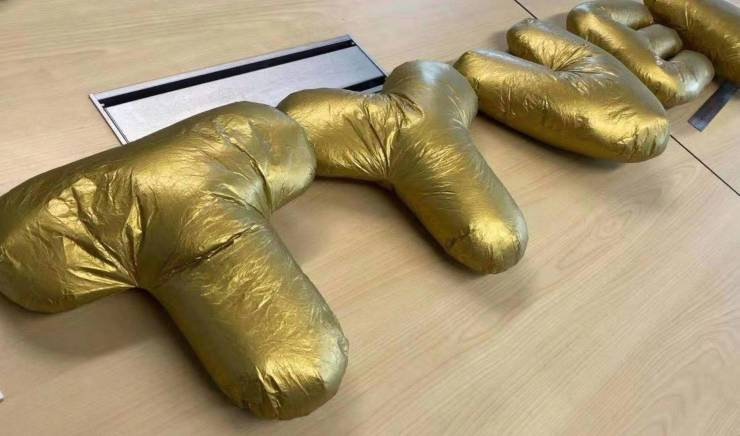4拼读游戏Spelling Game

（尺寸约：400*400*50，TYVEK5个字）5 我爱学习（销售产品）

（尺寸约：大300*400，中300*200，小250*100，3套）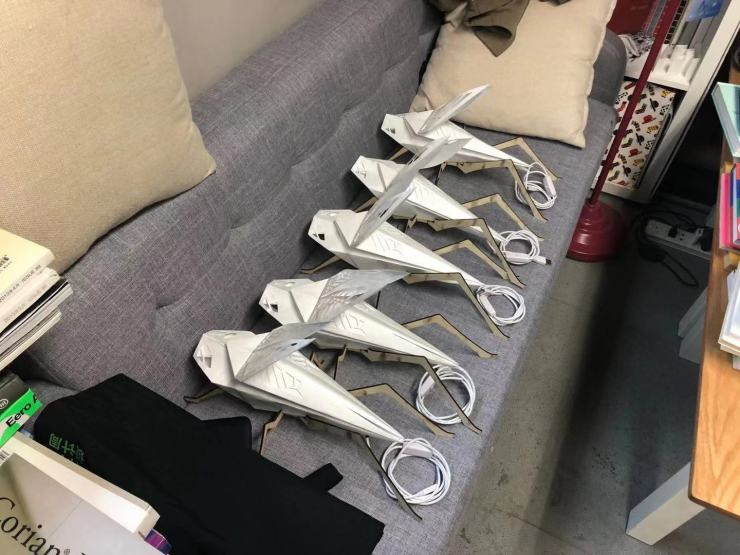6“小强”折纸灯" XIAOQIANG" origami lamp

（尺寸约：500*300*150，5个）

“小强”是基于儿童DIY的折纸灯具。木质插片组成的底座形成“小强”的6条腿，身体是由Tyvek采用折纸方式折叠而成，结合LED灯带形成最终的折纸灯具。DIY的方式满足了儿童爱动手的特点，“小强”的造型也激发儿童对于昆虫世界的兴趣和关注。

7“羽白”纸飞机 "YUBAI" paper plane

（尺寸约：300*220，3件）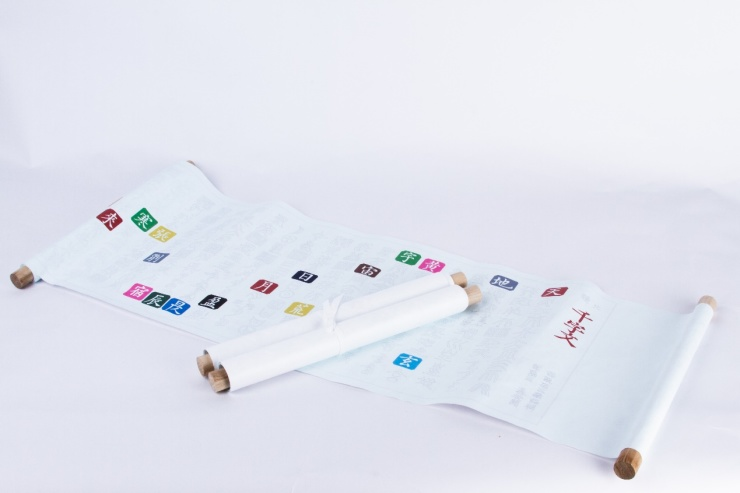8《2019千字文绘本》《2019Thousand Character Classic-picture book》

（销售产品）

（尺寸约：1000*300，3件，6000x300，1件供现场体验）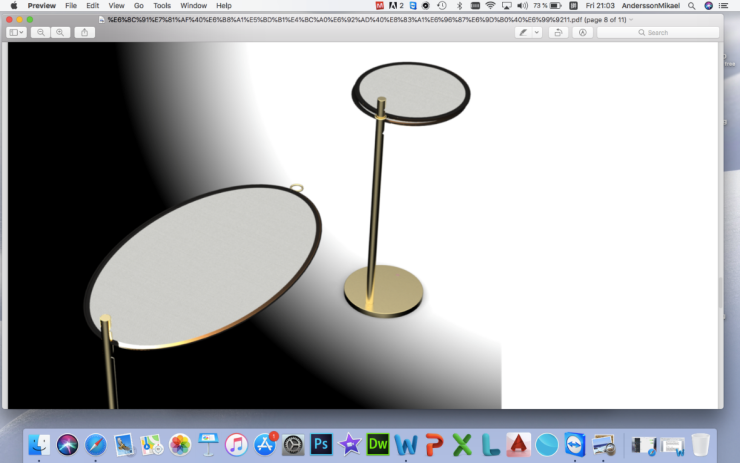9 VERY Light挑灯

（尺寸约：300*300*500，1件，需要电源）

Very light中文为“非常轻”，本设计是利用特卫强材料特性的“轻”，恰巧＂light”和“灯”也同英文，加之设计形态的特征，故得名:“挑灯”。挑灯，是为童阅读书写，设计的一款台灯或落地灯，特卫强轻盈，悬挑方式，可以获得更为宽阔的照射面积，使光线更为柔和，灯光可以从上部或下部，或同时照射特卫强的反射表面;亦可，将反光面折叠变为原来1/3的面积，再通过扣环或松紧带，将其固定在灯头，使其获得灯光的变化，并在运输或收纳时更为经济、友好。10梦兔 Dream Rabbit

（尺寸约：400*300*500，2个放在展柜上，2个从天花掉下，需要电源）

11蚕丝洞

（尺寸约：3000*2000*1500）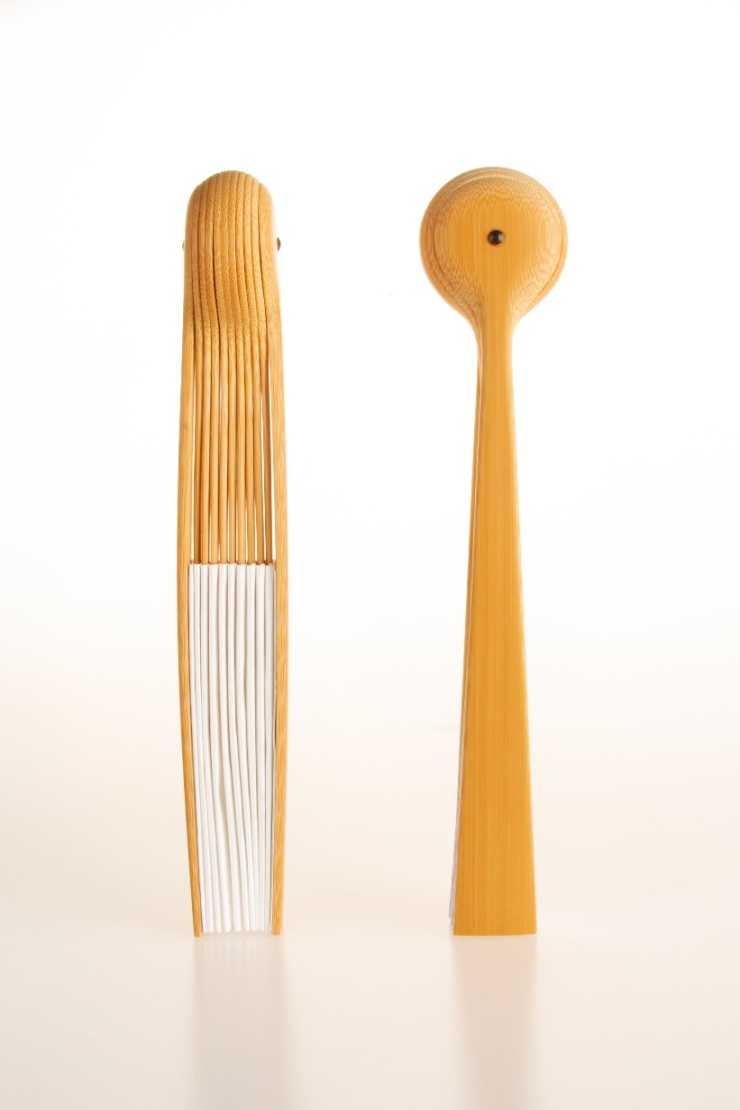12 强星人 Alien Johnny （销售产品）

（尺寸约：闭合180*33*22，打开250*180*22，3件）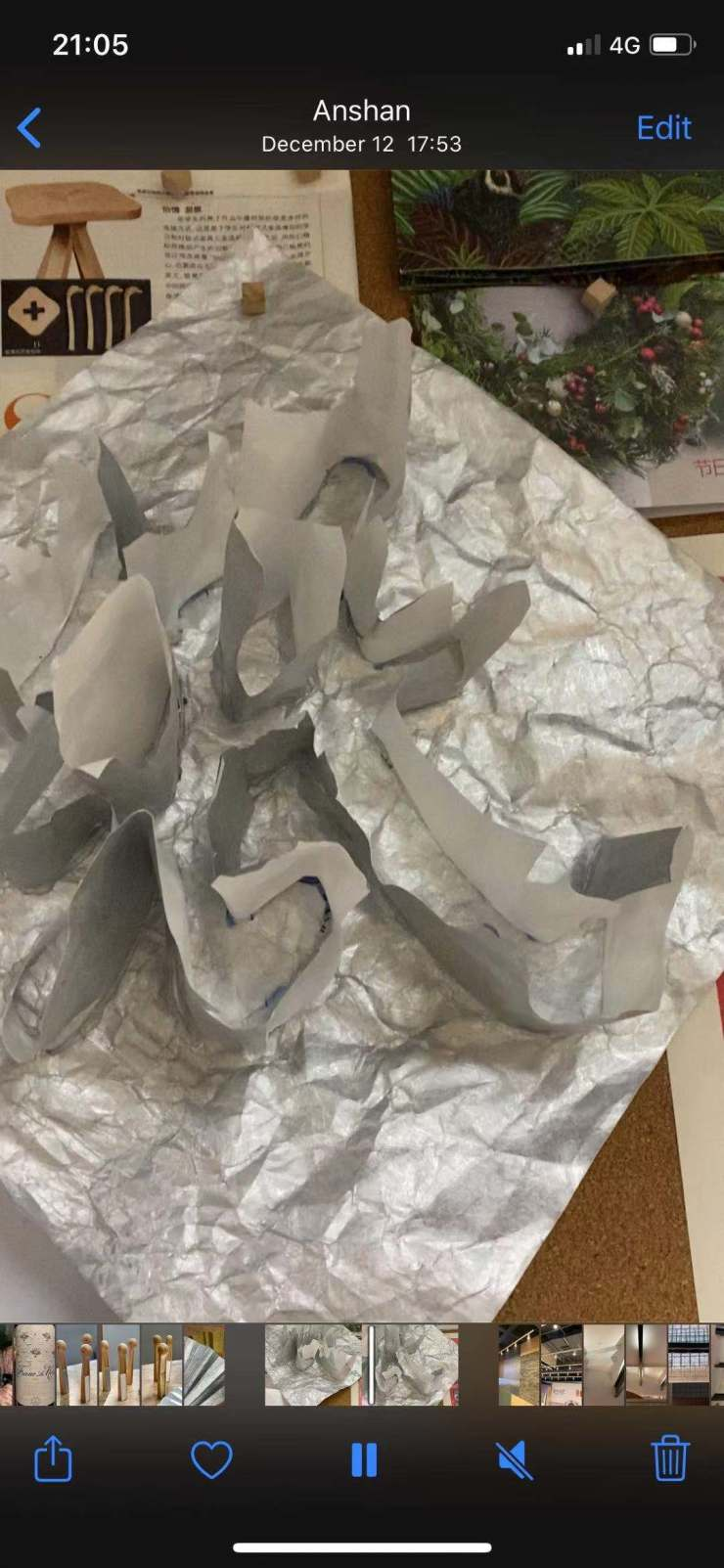13纸迷字谜 Text Fan, Text Maze

（尺寸约：1000*2000，3件，倒扣在灯带间）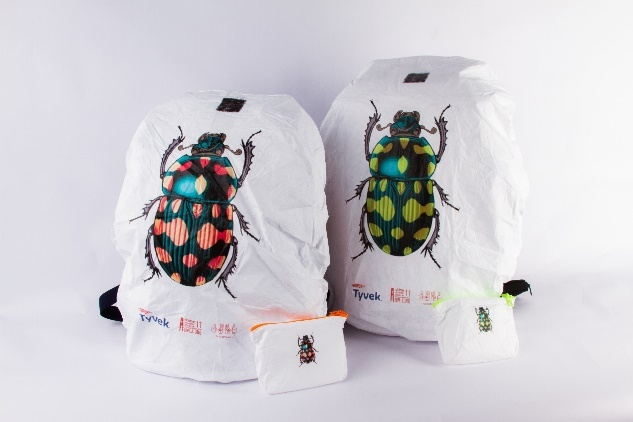14 小强护罩：Xiao Qiang Protection Shield （销售产品）

（尺寸约：300*200*400，橙3件，绿3件，需要陪2个书包）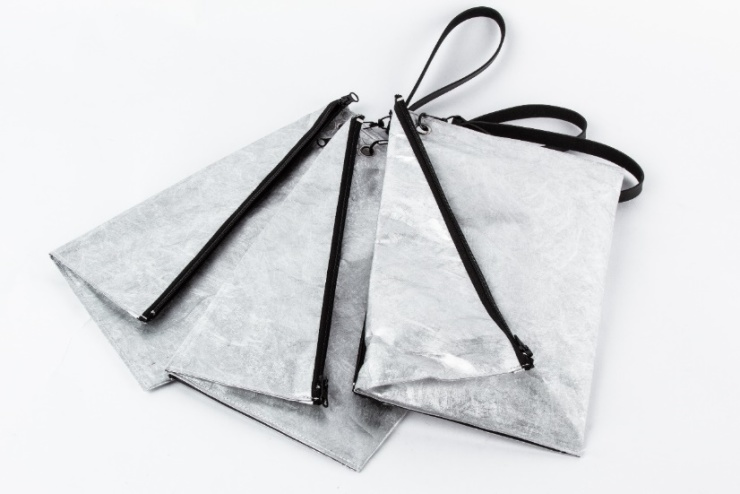15 折纸随身包

（尺寸约：300*200，3件 ）16阿强 Mr Qiang（销售产品）

（尺寸约：大1300*500*150，中500*300*150，小300*200*150，3套）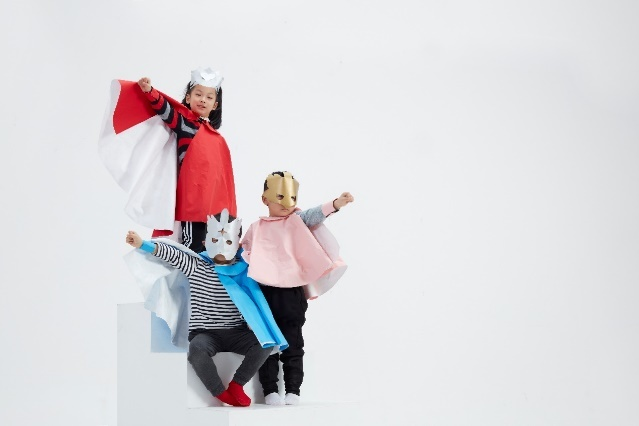17超级英雄SuperHeroes

（尺寸约：500*300*300）

Jean-Philippe BONZON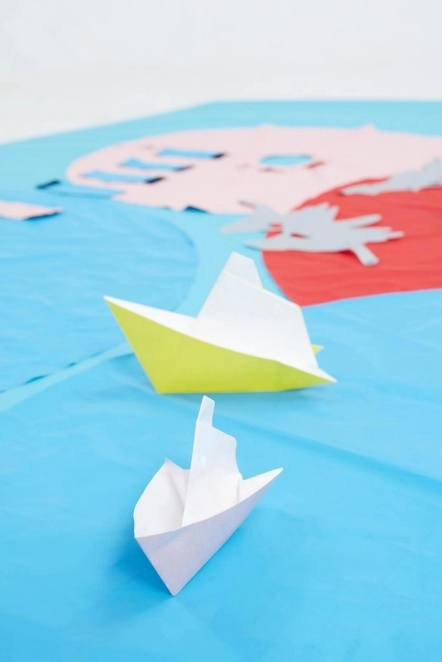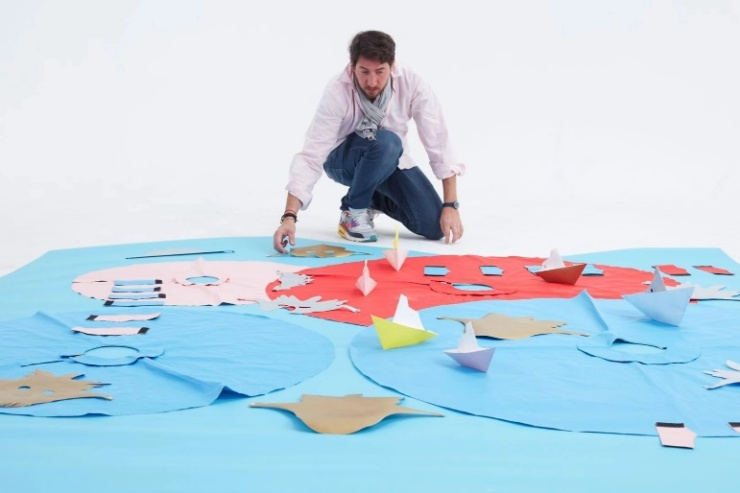18我的小船 My little boat

（尺寸约：100*30*50，3件，放在地上见图）

Jean-Philippe BONZON19展览馆 Exhibition hall

（尺寸约：1300*1000，1件，）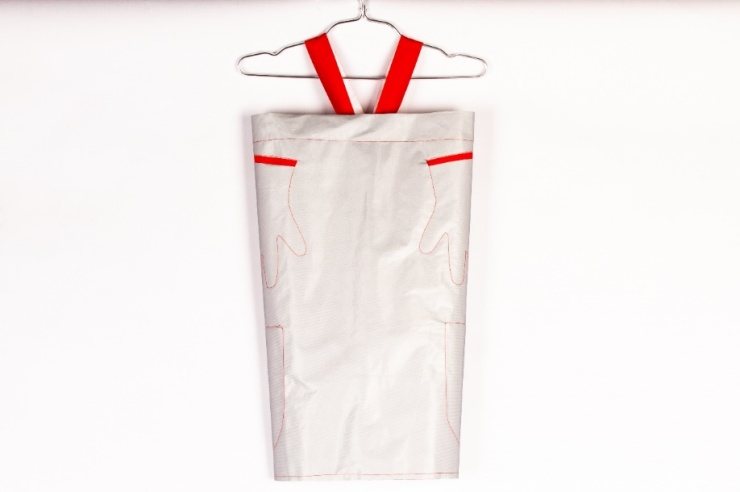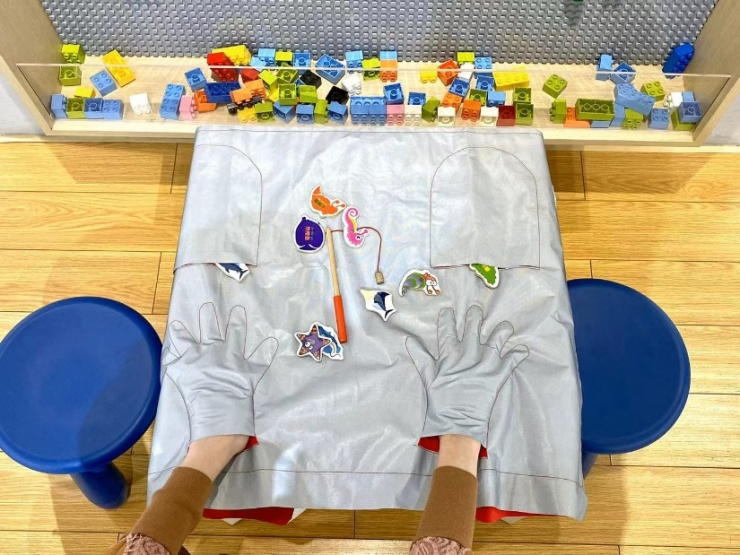20 知与行 Cognition and Behavior

（尺寸约：1000*500，1件）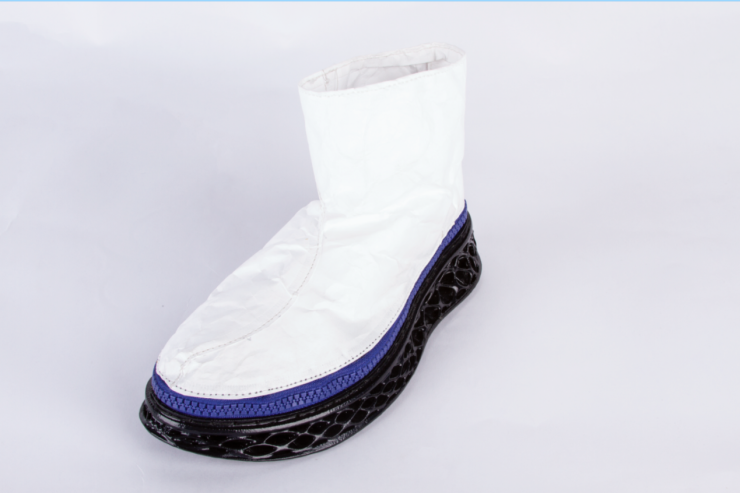21 X Baby

（尺寸约：350*150*200，1件）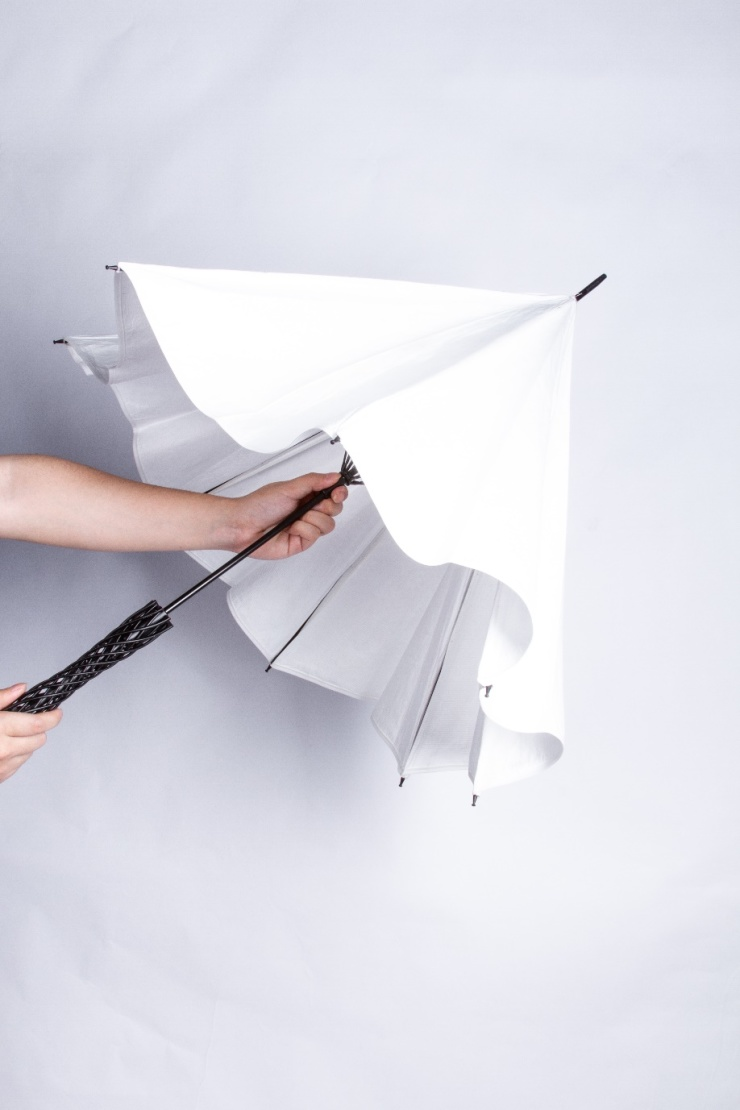22 Windrella

（尺寸约：1000*1000*800，1件 ）

Windrella是一款以风车为概念的趣味阳伞

23 坏脾气的鹅 Ornery Goose

（尺寸约：1000*1500，3件）

【关于杜邦】

【关于TX 淮海｜年轻力中心】

【设计师名录】

【嘉宾名录】

【主办单位】同济大学设计创意学院

【指导单位】上海工业设计协会、上海设计之都促进中心

【协办单位】杜邦中国集团有限公司、TX 淮海｜年轻力中心、中国工业设计博物馆 、上海国际工业设计中心、上海济裕国际贸易有限公司

【支持单位】内里百货、同济天地、渡影传播、阔合建筑、多少moreless原创家具

【媒体支持】上海电视台、Shanghai Daily、新民晚报、搜狐家居、理想家、澎湃（艺术）、优酷视频、网易家居、太平洋家居、腾讯家居、周末画报、解放日报（上海观察）

`声明：本文由入驻焦点开放平台的作者撰写，除焦点官方账号外，观点仅代表作者本人，不代表焦点立场错误信息举报电话： 400-099-0099，邮箱：jubao@vip.sohu.com，或点此进行意见反馈，或点此进行举报投诉。`A B C D E F G H J K L M N P Q R S T W X Y Z
A - B - C - D - E
• A
• 鞍山
• 安庆
• 安阳
• 安顺
• 安康
• 澳门
• B
• 北京
• 保定
• 包头
• 巴彦淖尔
• 本溪
• 蚌埠
• 亳州
• 滨州
• 北海
• 百色
• 巴中
• 毕节
• 保山
• 宝鸡
• 白银
• 巴州
• C
• 承德
• 沧州
• 长治
• 赤峰
• 朝阳
• 长春
• 常州
• 滁州
• 池州
• 长沙
• 常德
• 郴州
• 潮州
• 崇左
• 重庆
• 成都
• 楚雄
• 昌都
• 慈溪
• 常熟
• D
• 大同
• 大连
• 丹东
• 大庆
• 东营
• 德州
• 东莞
• 德阳
• 达州
• 大理
• 德宏
• 定西
• 儋州
• 东平
• E
• 鄂尔多斯
• 鄂州
• 恩施
F - G - H - I - J
• F
• 抚顺
• 阜新
• 阜阳
• 福州
• 抚州
• 佛山
• 防城港
• G
• 赣州
• 广州
• 桂林
• 贵港
• 广元
• 广安
• 贵阳
• 固原
• H
• 邯郸
• 衡水
• 呼和浩特
• 呼伦贝尔
• 葫芦岛
• 哈尔滨
• 黑河
• 淮安
• 杭州
• 湖州
• 合肥
• 淮南
• 淮北
• 黄山
• 菏泽
• 鹤壁
• 黄石
• 黄冈
• 衡阳
• 怀化
• 惠州
• 河源
• 贺州
• 河池
• 海口
• 红河
• 汉中
• 海东
• I
• J
• 晋中
• 锦州
• 吉林
• 鸡西
• 佳木斯
• 嘉兴
• 金华
• 景德镇
• 九江
• 吉安
• 济南
• 济宁
• 焦作
• 荆门
• 荆州
• 江门
• 揭阳
• 金昌
• 酒泉
• 嘉峪关
K - L - M - N - P
• K
• 开封
• 昆明
• 昆山
• L
• 廊坊
• 临汾
• 辽阳
• 连云港
• 丽水
• 六安
• 龙岩
• 莱芜
• 临沂
• 聊城
• 洛阳
• 漯河
• 娄底
• 柳州
• 来宾
• 泸州
• 乐山
• 六盘水
• 丽江
• 临沧
• 拉萨
• 林芝
• 兰州
• 陇南
• M
• 牡丹江
• 马鞍山
• 茂名
• 梅州
• 绵阳
• 眉山
• N
• 南京
• 南通
• 宁波
• 南平
• 宁德
• 南昌
• 南阳
• 南宁
• 内江
• 南充
• P
• 盘锦
• 莆田
• 平顶山
• 濮阳
• 攀枝花
• 普洱
• 平凉
Q - R - S - T - W
• Q
• 秦皇岛
• 齐齐哈尔
• 衢州
• 泉州
• 青岛
• 清远
• 钦州
• 黔南
• 曲靖
• 庆阳
• R
• 日照
• 日喀则
• S
• 石家庄
• 沈阳
• 双鸭山
• 绥化
• 上海
• 苏州
• 宿迁
• 绍兴
• 宿州
• 三明
• 上饶
• 三门峡
• 商丘
• 十堰
• 随州
• 邵阳
• 韶关
• 深圳
• 汕头
• 汕尾
• 三亚
• 三沙
• 遂宁
• 山南
• 商洛
• 石嘴山
• T
• 天津
• 唐山
• 太原
• 通辽
• 铁岭
• 泰州
• 台州
• 铜陵
• 泰安
• 铜仁
• 铜川
• 天水
• 天门
• W
• 乌海
• 乌兰察布
• 无锡
• 温州
• 芜湖
• 潍坊
• 威海
• 武汉
• 梧州
• 渭南
• 武威
• 吴忠
• 乌鲁木齐
X - Y - Z
• X
• 邢台
• 徐州
• 宣城
• 厦门
• 新乡
• 许昌
• 信阳
• 襄阳
• 孝感
• 咸宁
• 湘潭
• 湘西
• 西双版纳
• 西安
• 咸阳
• 西宁
• 仙桃
• 西昌
• Y
• 运城
• 营口
• 盐城
• 扬州
• 鹰潭
• 宜春
• 烟台
• 宜昌
• 岳阳
• 益阳
• 永州
• 阳江
• 云浮
• 玉林
• 宜宾
• 雅安
• 玉溪
• 延安
• 榆林
• 银川
• Z
• 张家口
• 镇江
• 舟山
• 漳州
• 淄博
• 枣庄
• 郑州
• 周口
• 驻马店
• 株洲
• 张家界
• 珠海
• 湛江
• 肇庆
• 中山
• 自贡
• 资阳
• 遵义
• 昭通
• 张掖
• 中卫

1室1厅1厨1卫1阳台

1
2
3
4
5

0
1
2

1

1

0
1
2
3报名成功，资料已提交审核A B C D E F G H J K L M N P Q R S T W X Y Z
A - B - C - D - E
• A
• 鞍山
• 安庆
• 安阳
• 安顺
• 安康
• 澳门
• B
• 北京
• 保定
• 包头
• 巴彦淖尔
• 本溪
• 蚌埠
• 亳州
• 滨州
• 北海
• 百色
• 巴中
• 毕节
• 保山
• 宝鸡
• 白银
• 巴州
• C
• 承德
• 沧州
• 长治
• 赤峰
• 朝阳
• 长春
• 常州
• 滁州
• 池州
• 长沙
• 常德
• 郴州
• 潮州
• 崇左
• 重庆
• 成都
• 楚雄
• 昌都
• 慈溪
• 常熟
• D
• 大同
• 大连
• 丹东
• 大庆
• 东营
• 德州
• 东莞
• 德阳
• 达州
• 大理
• 德宏
• 定西
• 儋州
• 东平
• E
• 鄂尔多斯
• 鄂州
• 恩施
F - G - H - I - J
• F
• 抚顺
• 阜新
• 阜阳
• 福州
• 抚州
• 佛山
• 防城港
• G
• 赣州
• 广州
• 桂林
• 贵港
• 广元
• 广安
• 贵阳
• 固原
• H
• 邯郸
• 衡水
• 呼和浩特
• 呼伦贝尔
• 葫芦岛
• 哈尔滨
• 黑河
• 淮安
• 杭州
• 湖州
• 合肥
• 淮南
• 淮北
• 黄山
• 菏泽
• 鹤壁
• 黄石
• 黄冈
• 衡阳
• 怀化
• 惠州
• 河源
• 贺州
• 河池
• 海口
• 红河
• 汉中
• 海东
• I
• J
• 晋中
• 锦州
• 吉林
• 鸡西
• 佳木斯
• 嘉兴
• 金华
• 景德镇
• 九江
• 吉安
• 济南
• 济宁
• 焦作
• 荆门
• 荆州
• 江门
• 揭阳
• 金昌
• 酒泉
• 嘉峪关
K - L - M - N - P
• K
• 开封
• 昆明
• 昆山
• L
• 廊坊
• 临汾
• 辽阳
• 连云港
• 丽水
• 六安
• 龙岩
• 莱芜
• 临沂
• 聊城
• 洛阳
• 漯河
• 娄底
• 柳州
• 来宾
• 泸州
• 乐山
• 六盘水
• 丽江
• 临沧
• 拉萨
• 林芝
• 兰州
• 陇南
• M
• 牡丹江
• 马鞍山
• 茂名
• 梅州
• 绵阳
• 眉山
• N
• 南京
• 南通
• 宁波
• 南平
• 宁德
• 南昌
• 南阳
• 南宁
• 内江
• 南充
• P
• 盘锦
• 莆田
• 平顶山
• 濮阳
• 攀枝花
• 普洱
• 平凉
Q - R - S - T - W
• Q
• 秦皇岛
• 齐齐哈尔
• 衢州
• 泉州
• 青岛
• 清远
• 钦州
• 黔南
• 曲靖
• 庆阳
• R
• 日照
• 日喀则
• S
• 石家庄
• 沈阳
• 双鸭山
• 绥化
• 上海
• 苏州
• 宿迁
• 绍兴
• 宿州
• 三明
• 上饶
• 三门峡
• 商丘
• 十堰
• 随州
• 邵阳
• 韶关
• 深圳
• 汕头
• 汕尾
• 三亚
• 三沙
• 遂宁
• 山南
• 商洛
• 石嘴山
• T
• 天津
• 唐山
• 太原
• 通辽
• 铁岭
• 泰州
• 台州
• 铜陵
• 泰安
• 铜仁
• 铜川
• 天水
• 天门
• W
• 乌海
• 乌兰察布
• 无锡
• 温州
• 芜湖
• 潍坊
• 威海
• 武汉
• 梧州
• 渭南
• 武威
• 吴忠
• 乌鲁木齐
X - Y - Z
• X
• 邢台
• 徐州
• 宣城
• 厦门
• 新乡
• 许昌
• 信阳
• 襄阳
• 孝感
• 咸宁
• 湘潭
• 湘西
• 西双版纳
• 西安
• 咸阳
• 西宁
• 仙桃
• 西昌
• Y
• 运城
• 营口
• 盐城
• 扬州
• 鹰潭
• 宜春
• 烟台
• 宜昌
• 岳阳
• 益阳
• 永州
• 阳江
• 云浮
• 玉林
• 宜宾
• 雅安
• 玉溪
• 延安
• 榆林
• 银川
• Z
• 张家口
• 镇江
• 舟山
• 漳州
• 淄博
• 枣庄
• 郑州
• 周口
• 驻马店
• 株洲
• 张家界
• 珠海
• 湛江
• 肇庆
• 中山
• 自贡
• 资阳
• 遵义
• 昭通
• 张掖
• 中卫• 手机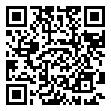• 分享
• 设计
免费设计
• 计算器
装修计算器
• 入驻
合作入驻
• 联系
联系我们
• 置顶
返回顶部